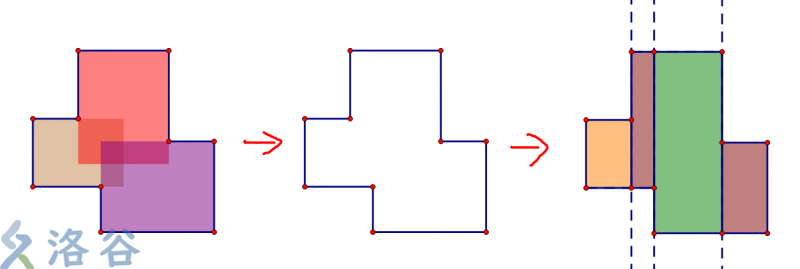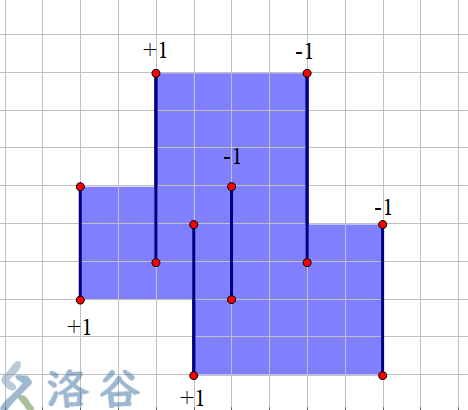# arrow_back线段树共44篇文章zc
2019-12-21 19:47:00zc
2019-12-21 19:47:00zc
2019-12-21 19:47:00zc
2019-12-21 19:47:00zc
2019-12-21 19:47:00

### 树状数组解法：zc
2019-12-21 19:47:00

f[i][k]为第i位,分成k

f[i][k] = MAX(f[j][k-1] + cnt[j+1][i])zc
2019-12-21 19:47:00zc
2019-12-21 19:47:00zc
2019-12-21 19:47:00zc
2019-12-21 19:47:00

$$\frac{1}{n}\sum_{i=1}^{n}(a_i-\bar a)^2 \\ = \frac 1n \sum_{i=1}^{n}(a_i^2-2\bar a\times a_i+{\bar a}^2) \\ = \frac 1n (\sum_{i=1}^nai^2 - 2\bar a\sum{i=1}^n a_i + n \bar a^2) \\ = \frac 1n \sum_{i=1}^n a_i^2 - \bar a^2$$

Search
search